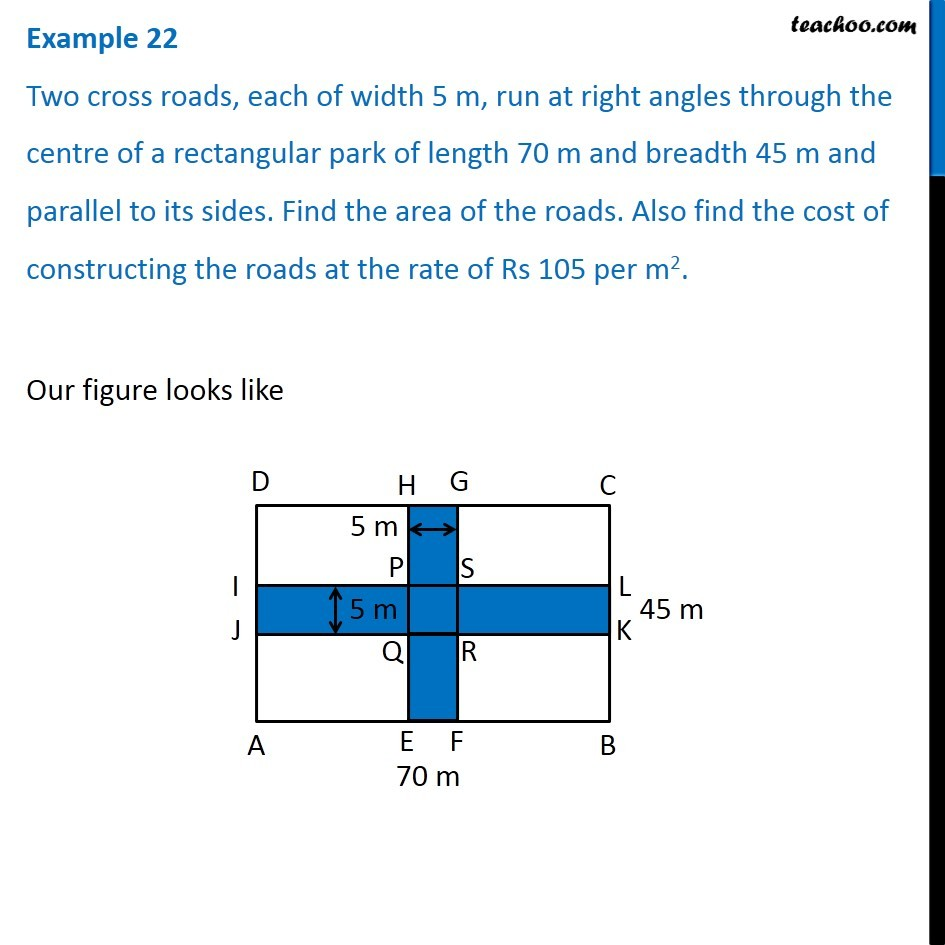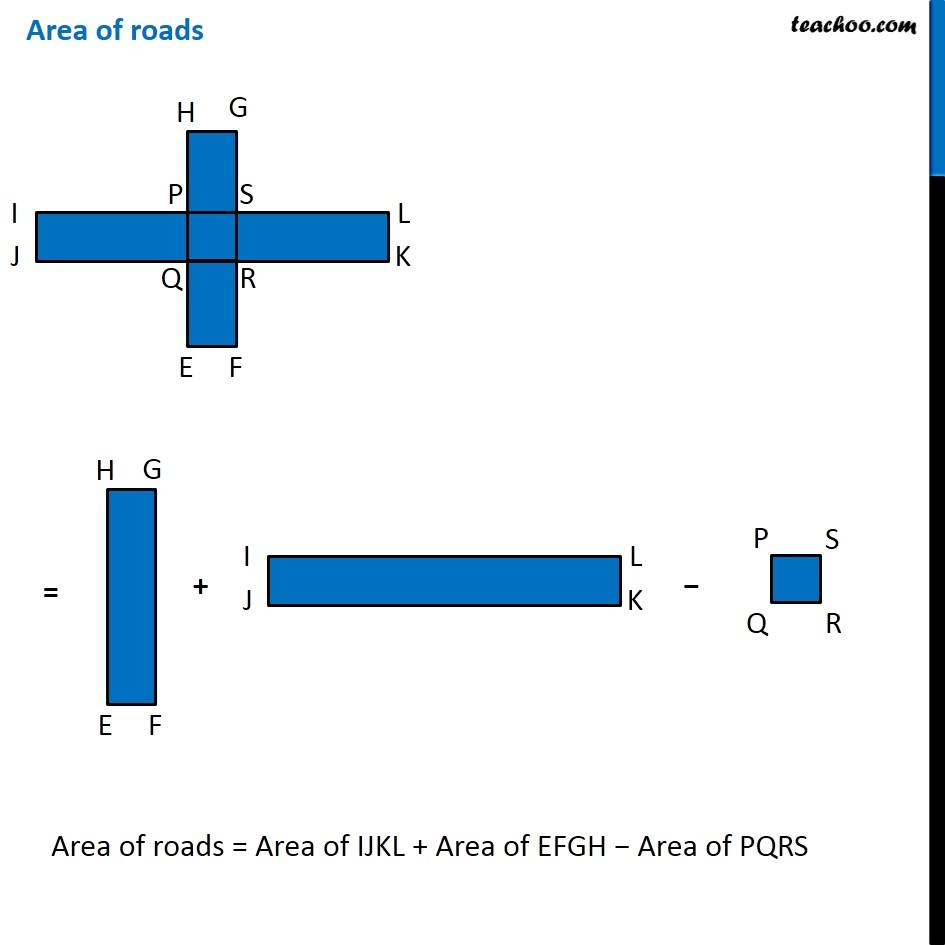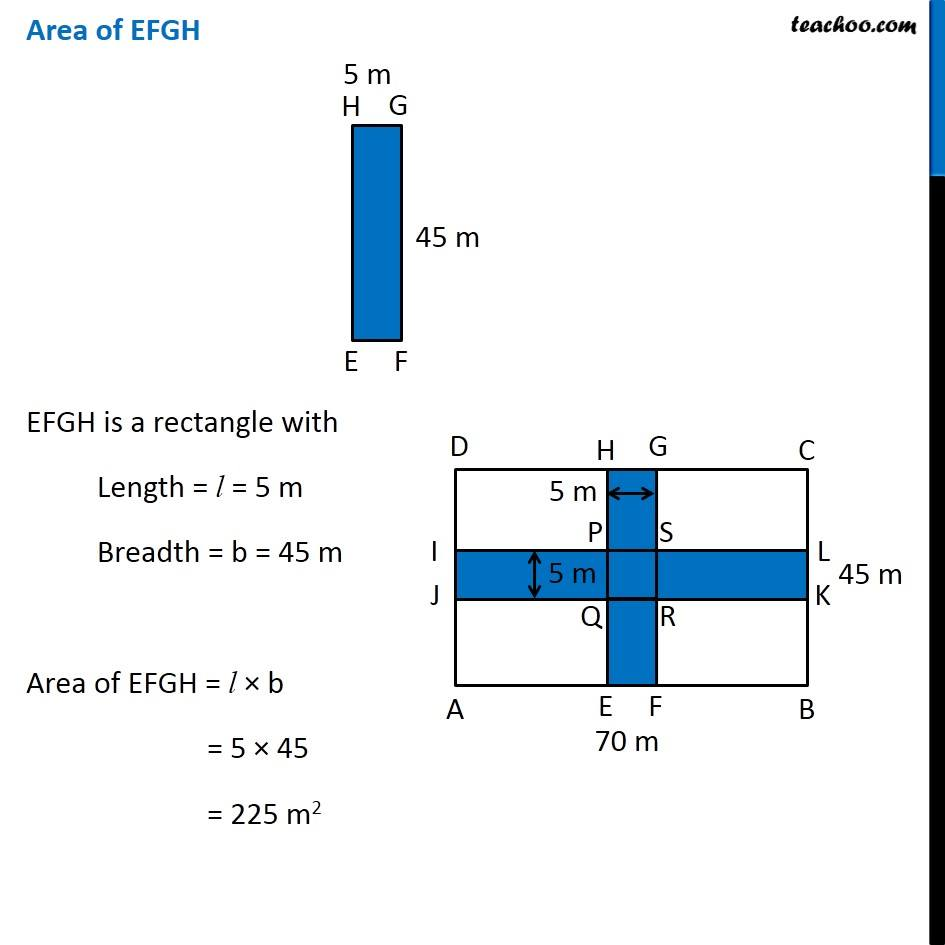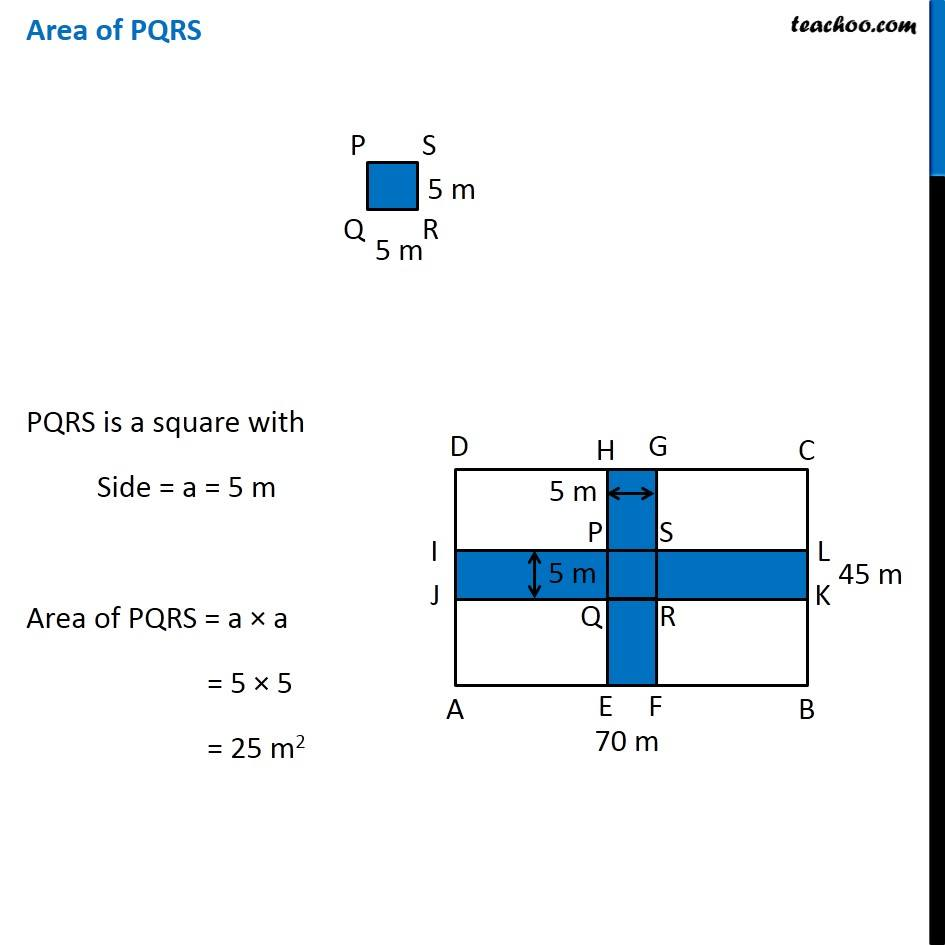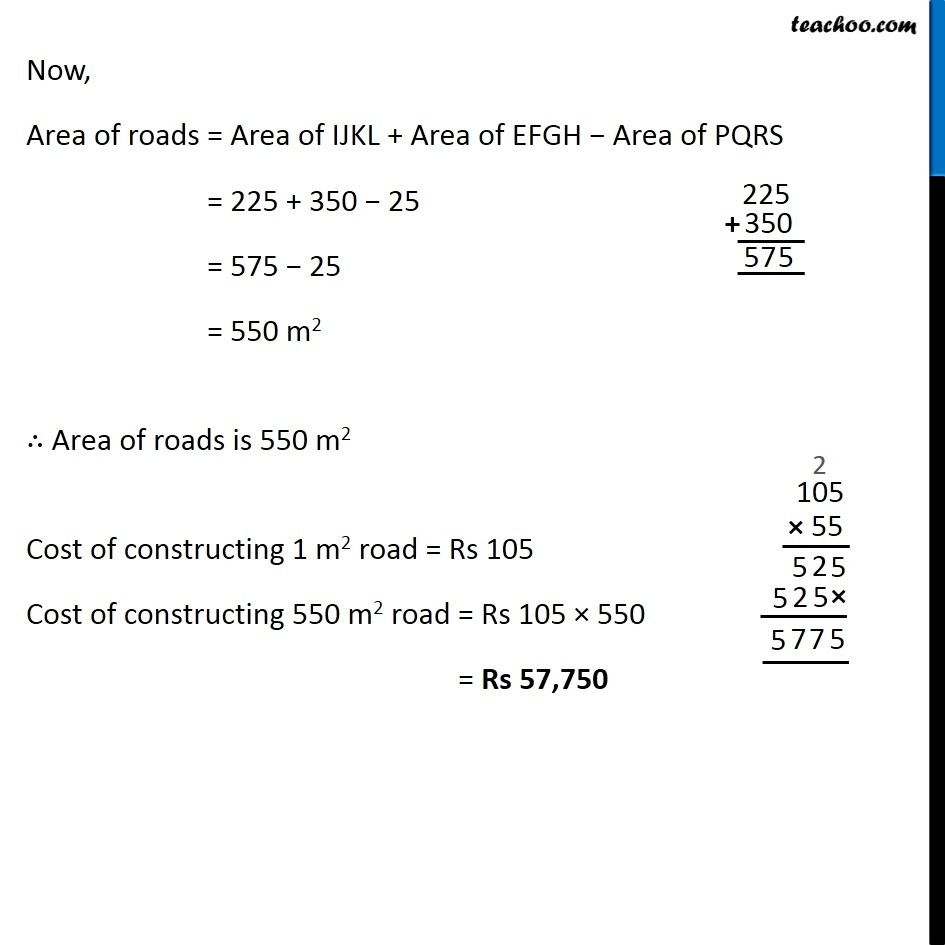1. Chapter 11 Class 7 Perimeter and Area
2. Serial order wise
3. Examples

Transcript

Example 22 Two cross roads, each of width 5 m, run at right angles through the centre of a rectangular park of length 70 m and breadth 45 m and parallel to its sides. Find the area of the roads. Also find the cost of constructing the roads at the rate of Rs 105 per m2. Our figure looks like Area of roads Area of roads = Area of IJKL + Area of EFGH − Area of PQRS Area of EFGH EFGH is a rectangle with Length = l = 5 m Breadth = b = 45 m Area of EFGH = l × b = 5 × 45 = 225 m2 Area of IJKL IJKL is a rectangle with Length = l = 70 m Breadth = b = 5 m Area of IJKL = l × b = 70 × 5 = 350 m2 Area of PQRS PQRS is a square with Side = a = 5 m Area of PQRS = a × a = 5 × 5 = 25 m2 Now, Area of roads = Area of IJKL + Area of EFGH − Area of PQRS = 225 + 350 − 25 = 575 − 25 = 550 m2 ∴ Area of roads is 550 m2 Cost of constructing 1 m2 road = Rs 105 Cost of constructing 550 m2 road = Rs 105 × 550 = Rs 57,750

Examples